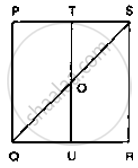# In the Below Figure, Pqrs is a Square and T and U Are Respectively, the Mid-points of Ps and Qr. Find the Area of δOts If Pq = 8 Cm. - Mathematics

In the below figure, PQRS is a square and T and U are respectively, the mid-points of PS
and QR. Find the area of ΔOTS if PQ = 8 cm.#### Solution

From the figure

T and U are the midpoints of PS and QR respectively.

∴ TU || PQ

⇒ TO || PQ

Thus, in ΔPQS,T is the midpoint of PS and TO || PQ

∴ TO = 1/2 PQ = 4cm

Also , TS = 1/2PS = 4cm

∴  ar (ΔOTS) =1/2 (TOxx TS) = 1/2 (4xx4) cm ^2  = 8 cm ^2

Concept: Corollary: A rectangle and a parallelogram on the same base and between the same parallels are equal in area.
Is there an error in this question or solution?

#### APPEARS IN

RD Sharma Mathematics for Class 9
Chapter 14 Areas of Parallelograms and Triangles
Exercise 14.3 | Q 2 | Page 44

Share